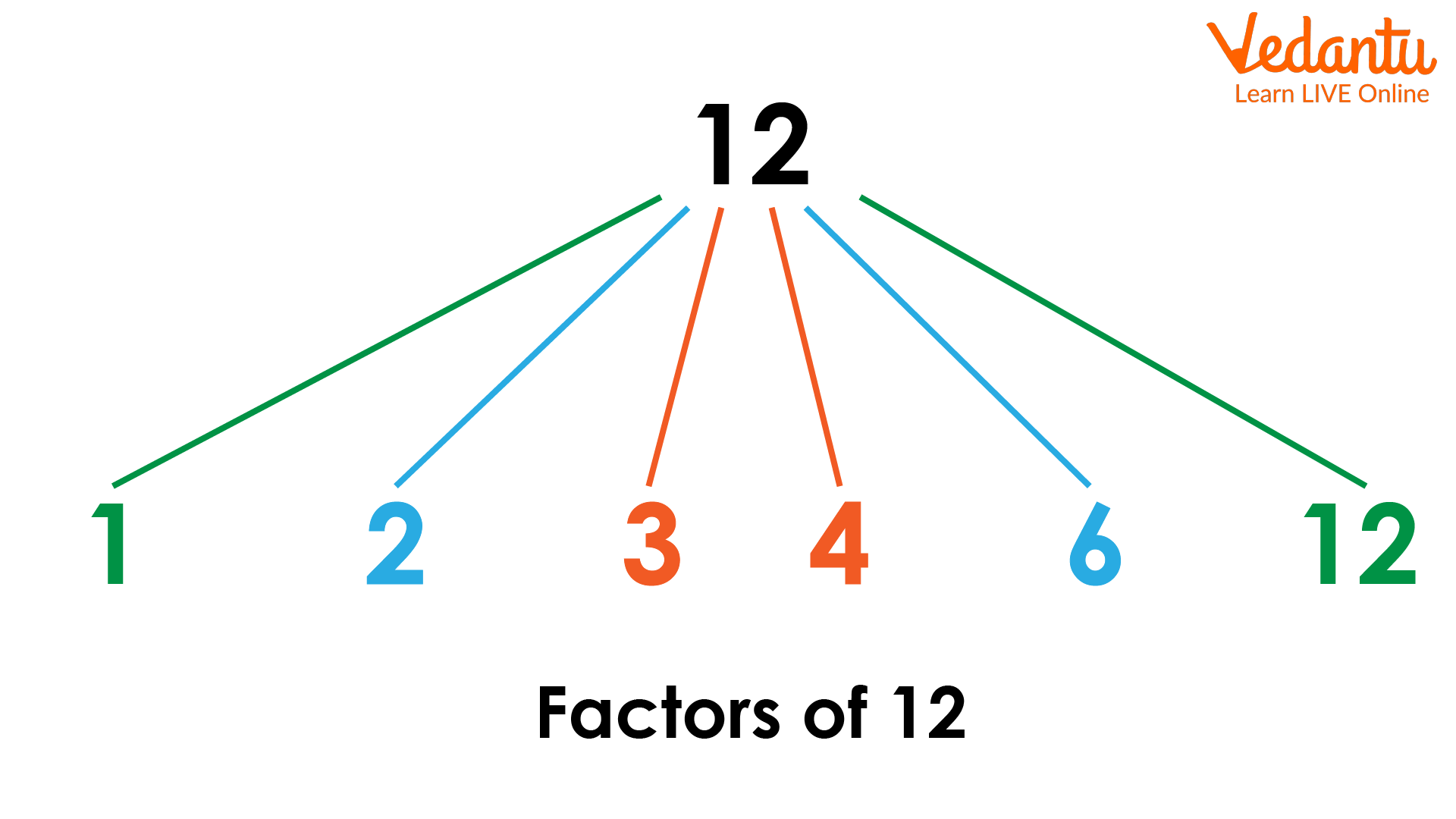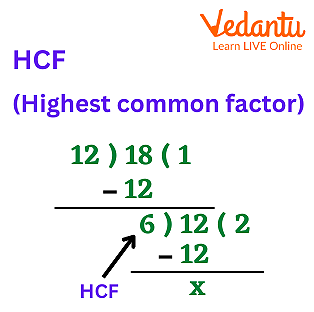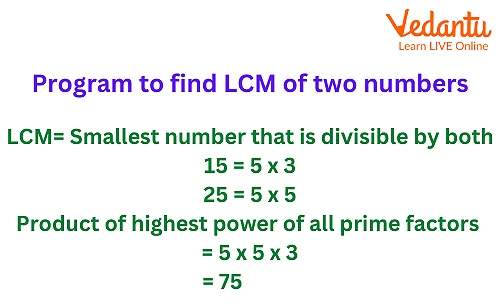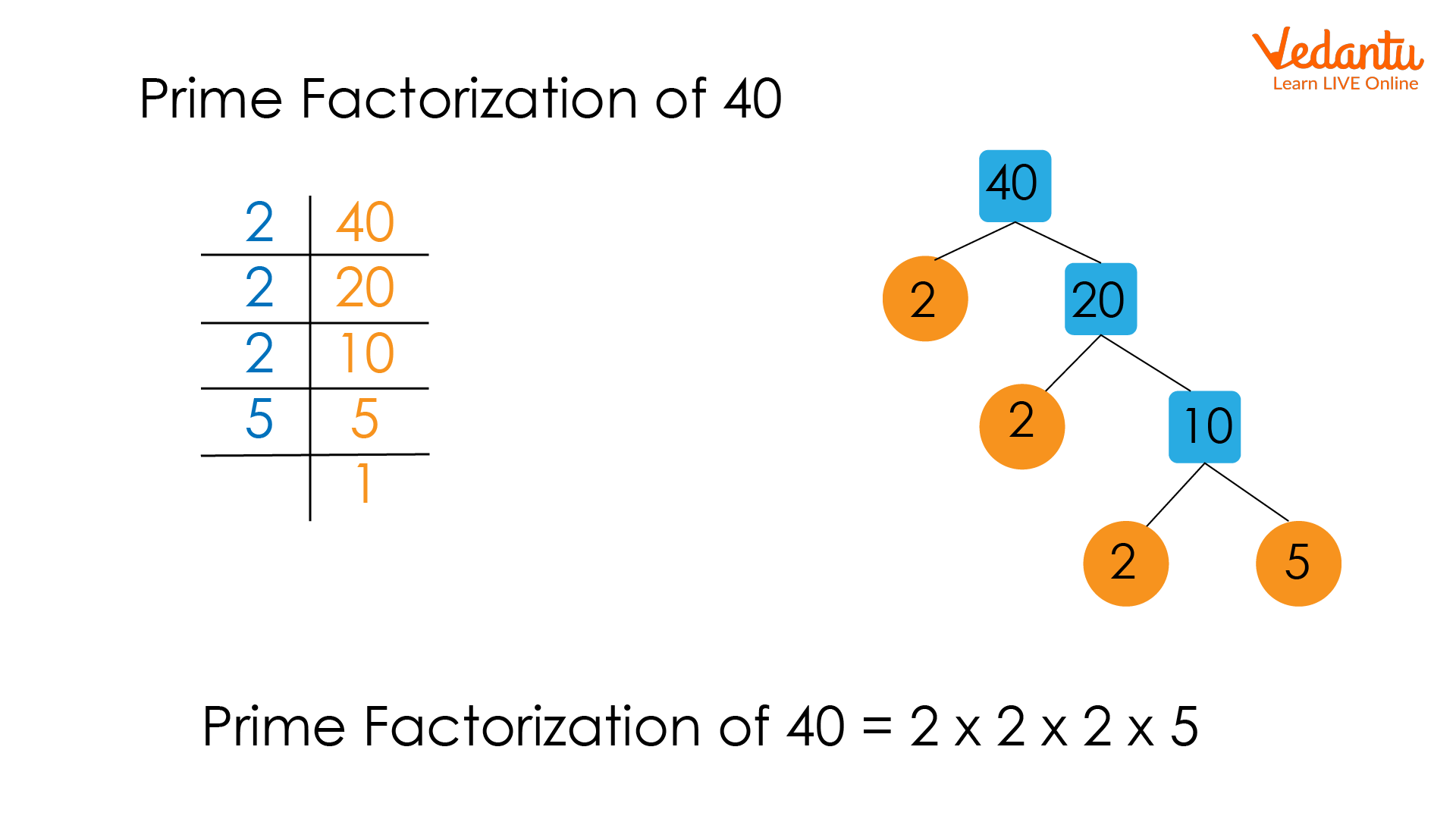Courses
Courses for Kids
Free study material
Offline Centres
More

# Factors ExamplesLast updated date: 05th Dec 2023
Total views: 102k
Views today: 1.02k## Introduction:

There is a number which divides with another number without leaving any reminder. We can find factors from two ways: multiplication and division. A number can be multiplied with another number to give a product so these numbers are called factors.

### Examples of Factors:

Let me have 10 mangoes and we have to divide that 10 mangoes between 10 people so that each person has an equal number of mangoes. So ,here $1 \times 10 = 10$ from this example we can conclude that one is a factor of 10. Now we decide to divide 10 mangoes between five people so that each person has an equal number of mangoes so we can give two mangoes to every person. Now $5 \times 2 = 10$. Now again we decide to share 10 mangoes between two people. Each person has an equal number of members so we can give five mangoes to every person now $2 \times 5 = 10$. So the factors of 10 are 1,2,5,10. So from this example we can conclude that when a number is divided by its factor then the remainder becomes zero.Factors of 12

### Common Factors:

Common factor is a number which is a factor of two or more numbers that implies two numbers that can be divided with the same number without leaving any reminder. 1 is the common factor to all the numbers. To find a common factor of two or more Numbers, firstly we have to write down factors of every number independently and after that we have to check which factors are common in both the numbers, the factors which are common in all the numbers are common factors.

Example: found out the common factors of 12, 18, 26, 30

Factors of 12 –1,2,3,4,6,12

Factors of 18–1,2,3,6,9,18

Factors of 26 –1,2,13,26

Factors of 30–1,2,3,5,6,10,15,30

Common factors of 12, 18, 26, 30 are 1 and 2.

### HCF:

HCF is called the highest common factor as its name suggests it is the highest common divisor of two or more numbers. Is also known as GCF, which is the greatest common factor. Now let’s discuss how to find HCF, so to find HCF of two or more number firstly we have to find factors of numbers separately and after that we have to find which factors are common after finding common factors we have to find which factories largest to all the numbers so the largest factor is called HCF.

Now let’s understand HCF with the help of an example. Let’s take two numbers 58 0 and 78 and find HCF.

Factors of 58 –1,2,29,58

Factors of 78 –1,2,3,6,13,26,39,78

Common factor of 58 and 78 –1,2

GCF of 58 and 78 is 2.HCF

### LCM:

It is the smallest number that is Multiple of two or more numbers. Let’s discuss LCM with the help of examples, take two numbers 3 and 5

Multiples of 3 are 3, 6, 9, 12, 15,…..

Multiples of 5 are 5,10,15,…..

Common numbers are 15,30,45,…..

The lease from all the common multiples of 3 and 5 is 15. Hence 15 is LCM of 3 and 5LCM

### Prime Factorisation:

When products of prime numbers are expressed as non prime numbers or composite numbers then this process is called prime factorisation. Now first discuss what prime numbers are: prime numbers are those numbers that only have two factors 1 and the number itself. Some prime numbers are 2,3,5,7,11,13,..so on. We can find prime factors with the help of tree method or division method. Let’s discuss some examples of prime factorisation

Prime factors of 12 –2x2x3

Prime factors of 36 –2x2x3x3Prime Factorization

### Solved Examples:

Q1. Find GCF of 28 and 34.

Solution: Factors of 28 – 1, 2, 4, 7, 14

Factors of 34–1, 2, 17, 34

Common factors of 28 and 34 –1,2

GCF of 28 and 34–2.

Q2. Find the LCM of 15 and 18

Solution:Multiples of 15 –15, 30, 45, 60, 75, 90, 105,…

Multiples of 18 –18, 36, 54, 72, 90,…..

So LCM of 15 and 18 is 90

Q3. Find the common factor 60 and 70

Solution:Factors of 60– 1,2,3,4,5,6,10,12,15,20,30,60

Factors of 70–1,2,5,7,10,35,70

Common factors of 60 and 70 are1,2,5,10

### Conclusion:

In this article we learned about factors with the help of examples. We also learned about HCF, LCM , prime factorisation, and common factors. We also learn about how does Numbers are interrelated to each other. Like without finding common factors we cannot find it safe for LCM.

## FAQs on Factors Examples

1. Why every Number can have at least two factors ?

Every number has at least two factors because every number must be divisible by 1 and the number itself.

2. Can factors of a number be greater than the number?

Factors of a number cannot be greater than the number because Factor of a number is just a divisor of that number so any divisor of a number cannot be greater than the number itself.

3. What are prime numbers?

Prime numbers are those numbers which are only divisible by 1 and the number itself.

4. What is GCF?

GCF is the greatest common factor as its name suggests it is the greatest common divisor of two or more numbers.

5. What is prime factorisation?

When any composite number is expressed as a product of two or more prime numbers this is called prime factorisation.